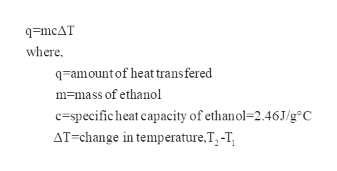# Calculate the amount of heat needed to boil149.gof ethanol (CH3CH2OH), beginning from a temperature of−89.9°C. Be sure your answer has a unit symbol and the correct number of significant digits.

Question
21 views

Calculate the amount of heat needed to boil

149.g

of ethanol (

CH3CH2OH

), beginning from a temperature of

−89.9°C

. Be sure your answer has a unit symbol and the correct number of significant digits.

check_circle

Step 1

Given:

Mass of ethanol=149.g

Temperature=-89.9oC

Step 2

Ethanol boiling point is 78.37oC.

The temperature change is given as

Step 3

To calculate amount of heat:

...help_outlineImage Transcriptioncloseq=mcAT where, q-amountof heat transfered m=mass of ethanol c=specific heat capacity of ethanol=2.46J/g°C AT=change in temperature,T, -T, fullscreen

### Want to see the full answer?

See Solution

#### Want to see this answer and more?

Solutions are written by subject experts who are available 24/7. Questions are typically answered within 1 hour.*

See Solution
*Response times may vary by subject and question.
Tagged in

### Physical Chemistry